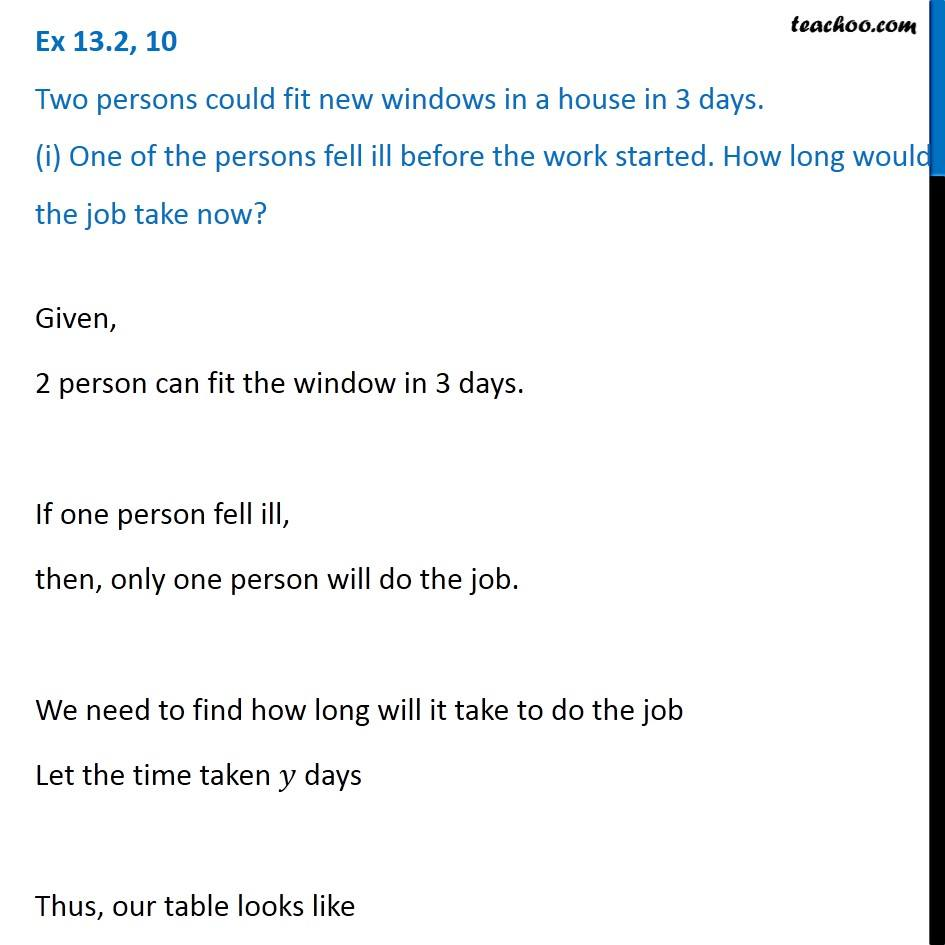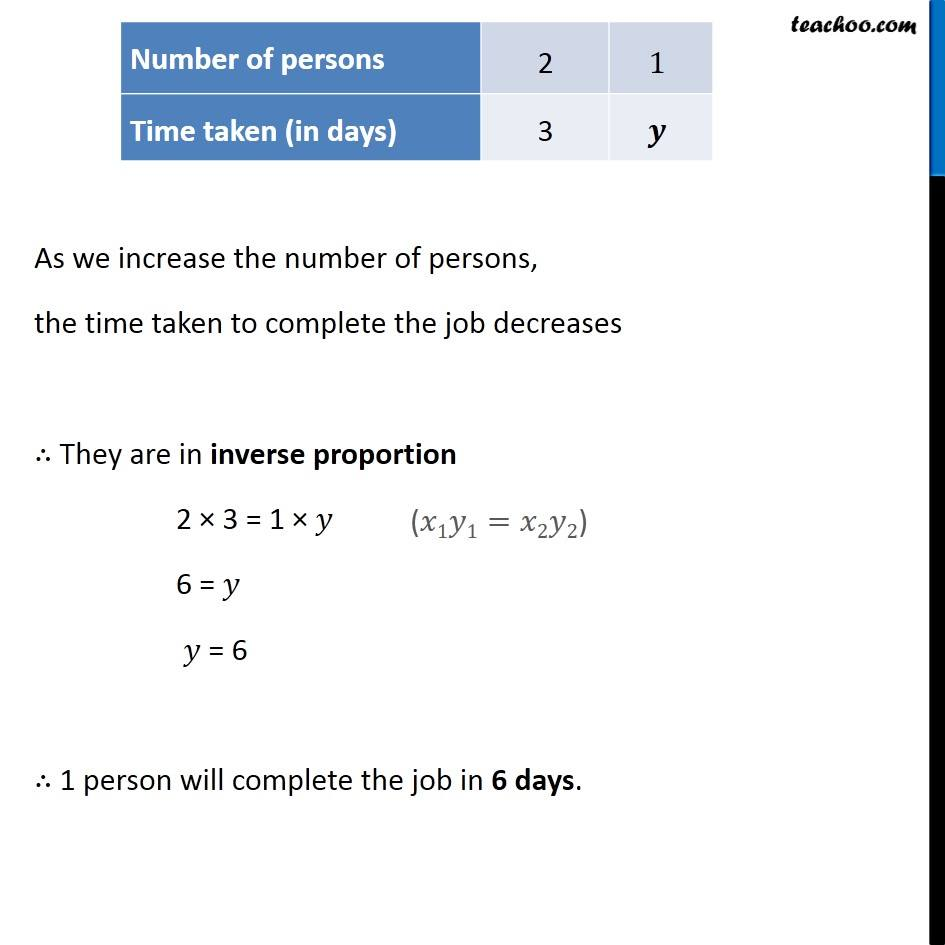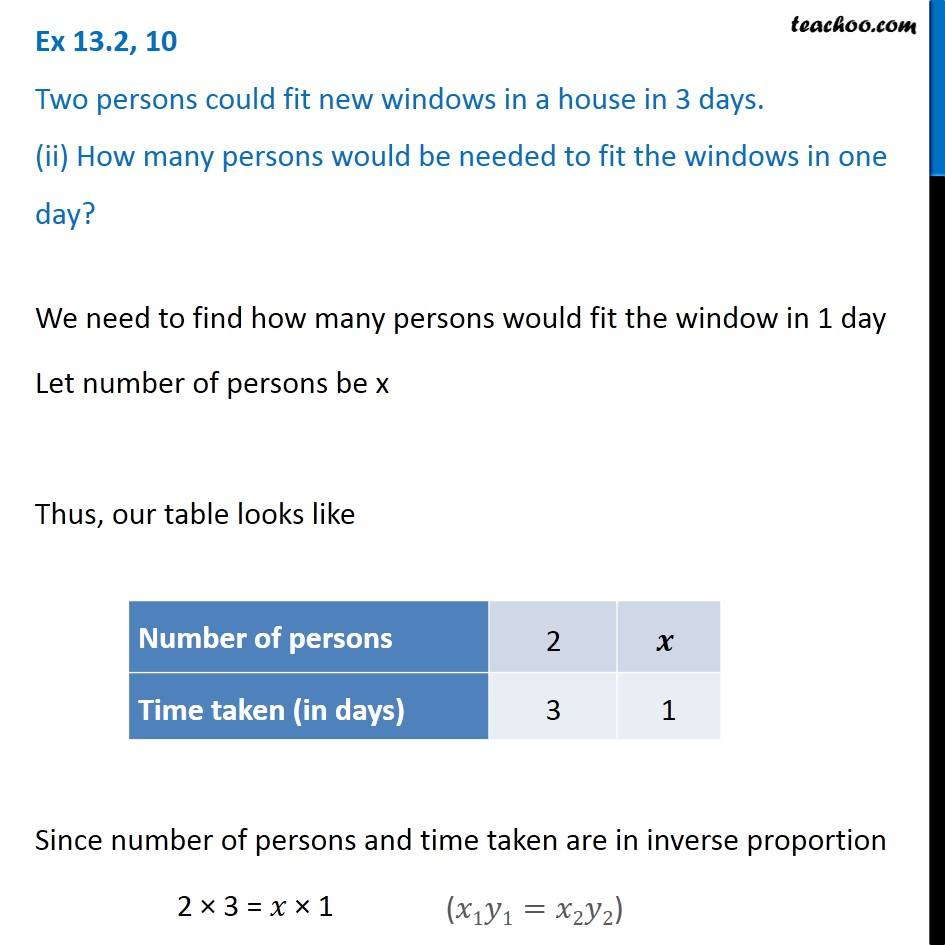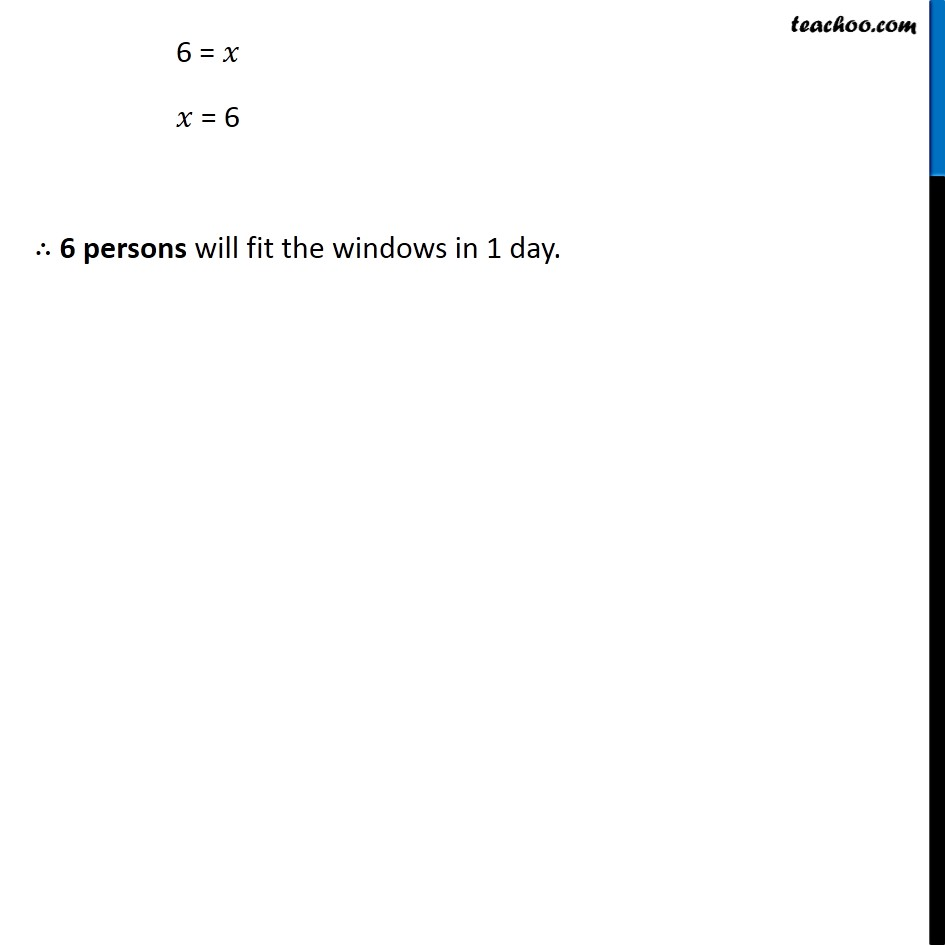Inverse Proportion

Chapter 13 Class 8 Direct and Inverse Proportions
Concept wise### Transcript

Ex 13.2, 10 Two persons could fit new windows in a house in 3 days. (i) One of the persons fell ill before the work started. How long would the job take now? Given, 2 person can fit the window in 3 days. If one person fell ill, then, only one person will do the job. We need to find how long will it take to do the job Let the time taken 𝑦 days Thus, our table looks like As we increase the number of persons, the time taken to complete the job decreases ∴ They are in inverse proportion 2 × 3 = 1 × 𝑦 6 = 𝑦 𝑦 = 6 ∴ 1 person will complete the job in 6 days. Ex 13.2, 10 Two persons could fit new windows in a house in 3 days. (ii) How many persons would be needed to fit the windows in one day? We need to find how many persons would fit the window in 1 day Let number of persons be x Thus, our table looks like Since number of persons and time taken are in inverse proportion 2 × 3 = 𝑥 × 1 6 = 𝑥 𝑥 = 6 ∴ 6 persons will fit the windows in 1 day.